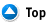Basic Shapes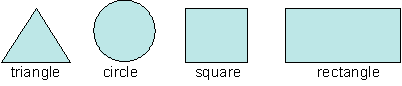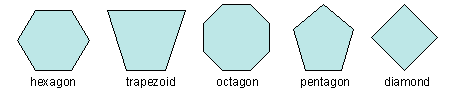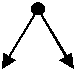acute angle An acute angle is an angle whose measure is greater than 0° and less than 90ş.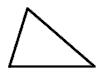acute triangle An acute triangle is a triangle that has three acute angles.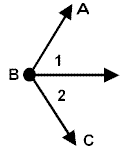<1 and <2 are adjacent<1 and

 Form of equation: Formula: Standard Form ax + by = c Slope Intercept Form/Y Intercept Form y = mx + b m = slopeb = y intercept X Intercept Form x = (y - b)/m b = y interceptm = slope Point-Slope Form y - y1 = m(x - x1) (x1,y1)m = slope